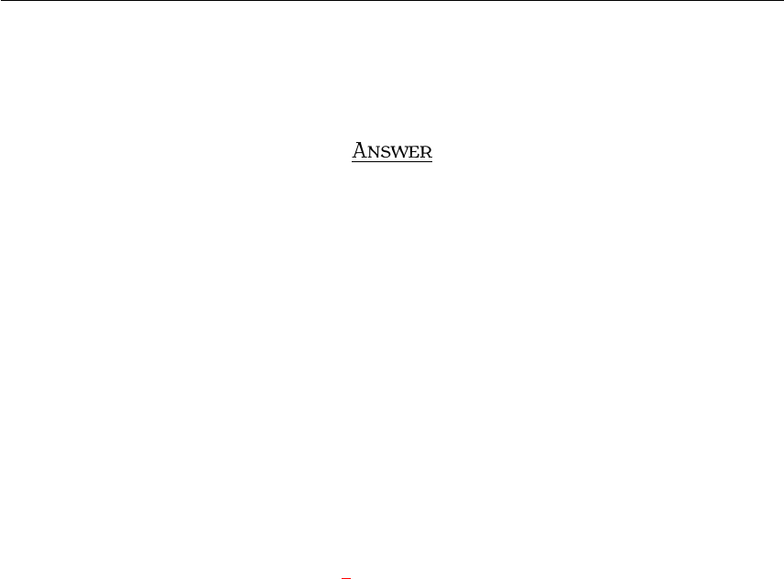Study Guides (400,000)
US (230,000)
BC (3,000)
MATH (90)
All (80)
Midterm

# Exam3210Sample2AnsExam

Department
Mathematics
Course Code
MATH 2211
Professor
All
Study Guide
Midterm

This preview shows pages 1-2. to view the full 6 pages of the document.MT T  S 
I S. Y
A , 
Q . THE PROPERTIES OF DETERMINANTS
Show that det(A) = 0 where
A=
0a b
a0c
bc0
A
Note that A=AT. Then we get det(A) = det((1)AT) = (1)3det(AT) = (1)3det(A) = det(A), i.e.,
2det(A) = 0 Ñdet(A) = 0. OR Calculate the determinant with respect to any column or any row of your
choice.
1

Only pages 1-2 are available for preview. Some parts have been intentionally blurred.Q . CRAMER’S RULE, VOLUME, AND LINEAR TRANSFORMATIONS
Find the inverse of the following matrix using inverse formula:
A=
1 2 3
0 2 3
0 0 3
.
A
Apply the Inverse Formula(see Example 3) given on page 203: We have det(A) = 6. We also have
C11 = (1)1+1det A11 = 6,
C21 = (1)2+1det A21 =6,
C31 = (1)3+1det A31 = 0,
C12 = (1)1+2det A12 = 0,
C22 = (1)2+2det A22 = 3,
C32 = (1)3+2det A32 =3,
C13 = (1)1+3det A13 = 0,
C23 = (1)2+3det A23 = 0,
C33 = (1)3+3det A33 = 2.
As a result, we obtain
A1=1
6
66 0
0 3 3
0 0 2
.
2